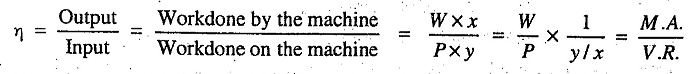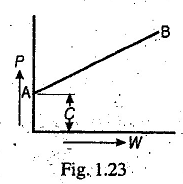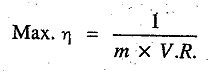### Lifting Machine (Lift)

Lift / Lifting machine - Mechanical advantage, Velocity Ratio, Efficiency, Reversible or non-Reversible machine.

It is a device, which enables us to lift a heavy load W, by a comparatively small effort P. The following terms are commonly used in lifting machines

1. Mechanical advantage (M.A.). It is the ratio of load lifted (W) to the effort applied (P).

2. Velocity ratio (V.R.). It is the ratio of the distance moved by the effort (y) to the distance moved by the load (x).

3. Input of the machine. It is the workdone on the machine. It is equal to the product of effort and the distance through which it moves (i.e. P x y).

4. Output of the machine. It is the workdone by the machine. It is equal to the product of load lifted and the distance through which it has been lifted (i.e. W x X).

5. Efficiency of the machine. It is ratio of output to the input of the machine. Mathematically, efficiency of the machine,6. Ideal machine. If the efficiency of the machine is 100 %, i.e. if output is equal to input, then the machine is said to be a perfect or ideal machine.

7. Reversible machine. If a machine is capable of doing some work in the reversed direction, after the effort is removed, then the machine is known as reversible machine. The condition for a machine to be reversible is that its efficiency should be more than 50 %.

8. Non- reversible or self locking machine. If a machine is not capable of doing some work in the reversed direction, after the effort is removed, then the machine is known as non-reversible or self locking machine. The condition for a machine to be non-reversible or self locking is that its efficiency should be less than 50 %.

9. Law of the machine. It is the relationship between the load lifted (W) and the effort applied (P). It is given by the equation,

P = m.W+C
where
m = A constant (called coefficient of friction) which is equal to the slope of the line AB as shown in Fig. 1.23, and
C = Another constant, which represents the machine friction.10. Maximum mechanical advantage. The maximum mechanical advantage of a lifting machine is given by
Max. M.A. = 1 / m

11. Maximum efficiency. The maximum efficiency of a lifting machine is given by1.### Are Engineers Favourite Among Girls?

What do girls think about men who are engineers and what type of engineers are most favourite among girls? A survey was conducted by a team of aboutmech with members chosen from different countries. Each team member carried out a neutral survey in his/ her country to evaluate what females think about most of the engineers from different engineering branches. An overview of different questions asked in survey is given below: Question: Which male engineers do you find more attractive as a husband? Almost 50% women voted for mechanical engineers to be the most attractive husbands among other engineers. So mechanical engineers are most favourite among girls as a husband. They find them more trustworthy, intelligent, and creative. A married women from California said, “If your husband is a mechanical engineer, he will help you in everyday tasks. Because mechanical engineers are good team players and they have creative ideas about everything.” Which male engineers do you find more